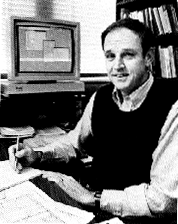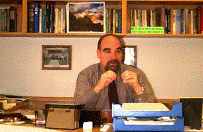Laboratory-Theory Interface: What Do Experiments Measure?

A. Instrumental responses are often highly precise

Major developments in experimental technology such as lasers, mass spectrometrometric tools, NMR spectrometers, and ultrafast pulse generating technologies have allowed us to make measurements that are extremely precise. For example, the microwave rotational spectra used earlier as an example can be performed with the absorption frequencies nk measured to better than one part in a million. Thus, if the theoretical equation relating the measured quantity to the desired molecular-level property (bond length in this example) is rigorously correct, the property can also be determined to high accuracy.

B. The relations between experimental signals and molecular quantities often are imprecise

However, as we saw earlier, the equations relating the experimental observations to the molecular level often suffer either because: (a) they have not (yet) been derived in a rigorous enough manner to render them adequately accurate or (b) they may involve concepts (e.g., bond length) that are not uniquely defined. Let us consider the implications of both of these deficiencies.

An example of the former shortcoming is provided by modern day enhancements to Eyring's expression for the rate coefficient:

ln (k) = A - Ea/RT.

Even years after Eyring showed how to relate A and Ea to molecule-level quantities, it had not been rigorously shown that the above expression was fully correct. In recent years, much effort has been devoted to deriving from first principles a correct expression for the temperature dependence of the rate coefficient. At present, the most advanced, reliable, and widely used versions of this theory can be derived most rigorously by considering so-called time correlation functions (W. H. Miller, B. Berne, and D. Chandler). Using ideas that date back to the early work of Wigner and Hirschfelder, these time correlation function expressions for the rates of chemical reaction can be extended to derive equations that are called (D. G. Truhlar) variational transition state theory (VTST) and other variants. Such theories account for tunneling through classically forbidden regions of the reactive potential energy surface near the transition state and for the fact that the "point of no return" on the reaction path may not occur precisely at the pass or coll on the energy surface. Nevertheless, even today there remain approximations and assumptions within the derivation of VTST that render the connection between the raw experimental rate data and the molecule- level quantities approximate (albeit to a higher level than in the times of Arrhenius or Eyring).Professor William MillerProfessor Bruce BerneProfessor David Chandler

In the author's opinion, the best example of the second shortcoming mentioned above remains the bond length concept that has already been discussed in some detail. In addition to the average of r-2 that results from microwave data and the average of r that can be extracted from dipole moment data on highly ionic molecules, there are yet other distinct values for bond lengths that result from x-ray, electron diffraction, and NMR experimental data.

In the former case, the scattering of the x-radiation is dominated by the electrons within the molecules. Although the molecule's electron density may be approximated by the sum of electron densities of its constituent atoms (each of which is centered on its own atomic center), the deformation of the latter density induced by bond formation can be substantial. As a result, the total electron density may not be adequately represented by sums of densities on atomic centers, and thus the scattered and subsequently diffracted x-ray photons may not produce patterns that measure spacings between these atomic centers.

In the electron diffraction case, the scattering of the electron beam is more strongly influenced by scattering from the nuclei than by the electrons. As a result, the diffraction pattern produced by the scattered electron beam produces a more direct probe of the distances between the nuclei.

Finally, nuclear magnetic resonance measurements of coupling constants between nearby magnetic nuclei can also be used to produce information about the interatomic distances. However, because the coupling constant between pairs of nuclei separated by a distance r contain contributions that vary as r-3 and as r-6 , the coupling constant extracted from experimental data produces only a vibrationally averaged value for r-3 and r-6 , not of r-2 nor of r.

In the sense detailed in the above discussion, it is therefore proper to say that the concept of bond length is not precisely defined so no improvements in experimental precision can help measure bond lengths to greater and greater accuracy.

#### C. Why Can Experiments Not Directly Determine Molecular Properties?

1. Experiments usually provide quantum-level data

Spectroscopic and diffraction experiments, in particular, interrogate the molecular sample in a quantum-state manner. For example, the vibrations of the OCS molecule are observed in an infrared absorption experiment which determines the energy differences between neighboring vibrational energy states DE (ni Æ ni+1). It is only through using a theoretical model such as the harmonic oscillator energy expression

E = S i=1,3N-6 hwi (ni+1/2)

or the Morse oscillator expression

E = S i=1,3N-6 hwi [(ni+1/2) - (ni+1/2)2hwi/4De,i],

where De,i is the dissociation energy for the ith mode, that one can extract from measured DE values the freqencies wi that characterize the molecule's vibrational motions.

#### 2. Our concepts and molecular models are often cast in classical language

In contrast to the quantum state energy difference information that spectroscopic experiments usually provide, the models and pictures that we chemists use to describe molecules are cast in the language of classical mechanics. For example, the frequencies wi obtained from infrared data are usually viewed as the frequencies with which the molecule's bonds (lengths and angles) move. We view the distances [<v| r-2 |v> ]-1/2 obtained from microwave data as distances between atomic centers within a molecule. Such interpretations are only valid to the extent that we trust the equations (e.g., harmonic or Morse energy expressions or rigid-rotor energy formula) that connect the data to the model and as long as we realize that some of our concepts may be ambiguous when examined with finer and finer scrutiny.